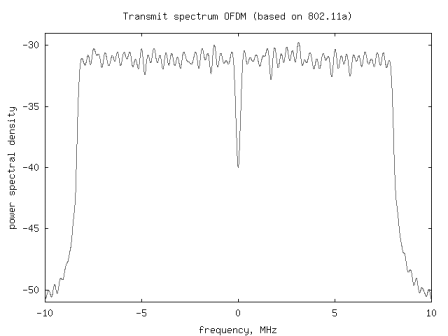- DSP log - http://www.dsplog.com -

Understanding an OFDM transmission

Posted By Krishna Sankar On February 3, 2008 @ 7:37 pm In OFDM | 201 Comments

Let us try to understand simulation of a typical Orthogonal Frequency Division Multiplexing (OFDM) transmission defined per IEEE 802.11a specification .

Orthogonal pulses
In a previous post (here ), we have understood that the minimum frequency separation for two sinusoidals with arbitrary phases to be orthogonal is , where is the symbol period.

In Orthogonal Frequency Division Multiplexing, multiple sinusoidals with frequency separation is used. The sinusoidals used in OFDM can be defined as (Refer Sec6.4.2 in [DIG-COMM-BARRY-LEE-MESSERSCHMITT ]:

, where

correspond to the frequency of the sinusoidal and

is a rectangular window over .

Using of inverse Fourier Transform

We now have understood that OFDM uses multiple sinusoidals having frequency separation where each sinusoidal gets modulated by independent information. The information is multiplied by the corresponding carrier and the sum of such modulated sinusoidals form the transmit signals. Mathematically, the transmit signal is,

The interpretation of the above equation is as follows:
(a) Each information signal multiplies the sinusoidal having frequency of .
(b) Sum of all such modulated sinusoidals are added and the resultant signal is sent out as .

Update: 29th May 2008

(thanks to the comment by Mr. Mork )

The sampled version of the above equation is,

It is reasonable to understand that above operation corresponds to an inverse Discrete Fourier Transform (IDFT) operation.

Understanding of an OFDM transmission specified per IEEE 802.11a [80211A ]

From the Table 79 in [80211A ], the symbol duration .
This implies that the used subcarriers are and so on.
The available bandwidth of 20MHz is split into 64 subcarriers. Out of the available 64 subcarriers having indices , the number of used subcarriers is 52. The used subcarriers are having indices from are used for transmitting information sequence to .

To understand in detail the correspondence between the subcarrier index and frequency, one may refer to a previous post (here ).

Once the bits in each symbol are assigned to appropriate IDFT inputs (Ref. Figure 109 in [80211A ]), the IDFT operation is performed to obtained the time domain signal.

For each symbol, some samples from the end are appended to the beginning of the symbol (referred to as cyclic prefix insertion). We may discuss the pros/cons of cyclic prefix in a future post.

Simulation Model
A simple Octave script where a BPSK modulated signal is transmitted on the 52 used subcarriers, may be used for understanding generation of an OFDM signal. The script is loosely based on 802.11a specification .Figure: Spectrum of an OFDM transmission (based on 802.11a specification)

Reference

[DIG-COMM-BARRY-LEE-MESSERSCHMITT]Digital Communication: Third Edition, by John R. Barry, Edward A. Lee, David G. MesserschmittHope this helps.
Krishna

URL to article: http://www.dsplog.com/2008/02/03/understanding-an-ofdm-transmission/

URLs in this post:

 here : http://www.dsplog.com/2007/12/31/minimum-frequency-spacing-for-having-orthogonal-sinusoidals/